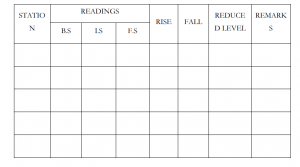# DIFFERENTIAL OR FLY LEVELLING (RISE AND FALL METHOD)

## DIFFERENTIAL OR FLY LEVELLING (RISE AND FALL METHOD)

In this post, I am going to discuss about to find the difference in elevation and to calculate the reduced level of various points by Rise and Fall method.

### Equipment used for fly levelling ( Rise and Fall method)

The equipment or instruments used for differential levelling are

Dumpy level, Leveling staff.

## Procedure for differential levelling using rise and fall method

The field procedure for differential levelling using rise and fall method and booking of staff reading is done in the same way as explained in the height of instrument method (each reading is entered on a different line in the appropriate column, except at a change point, where a FS and BS occupy the same line). However, the data booking is performed as shown in the table below.

TabulationCalculations

1. The difference in elevation between any two successive points (say A and B) can be calculated as:

Elevation difference between A and B = first reading at A – second reading at B

2. NOTE, for any two successive staff readings:
 Second reading smaller than first reading represents a Rise (The sign of elevation difference is positive).
 Second reading greater than first reading represents a Fall (The sign of elevation difference is negative).

3. If the elevation of the first point is known, then the elevation of the second point
can be calculated as:

Height of B = height of A + rise (in rise case)
or
Height of B = Height of A – fall (in fall case)

4. Checks: The following checks on the booking and arithmetic calculations are
performed: